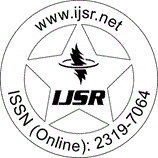International Journal of Science and Research (IJSR)
Call for Papers | Fully Refereed | Open Access | Double Blind Peer Reviewed

Review Papers | Electronics & Communication Engineering | India | Volume 3 Issue 3, March 2014

# Area Efficient Architecture for Convolution Using Vedic Mathematics

Karishma P Dighorikar | S. L. Haridas

Abstract: Convolution is a formal mathematical operation, just as multiplication, addition, and integration. Addition takes two numbers and produces a third number, while convolution takes two signals and produces a third signal. Convolution is used in the mathematics of many fields, such as probability and statistics. In linear systems, convolution is used to describe the relationship between three signals of interest: the input signal, the impulse response, and the output signal. Vedic mathematics is used since it reduces time, increases speeed and is easy to implement. It helps to solve problem 10-15 times faster. It covers explanation of several mathematical terms such as algebra, arithmetic, geometry etcThis paper presents a direct method of reducing convolution processing time using hardware computing and implementations of discrete linear convolution of two finite length sequences (NXN) The purpose of this research is to prove the feasibility of an application specific integrated circuit (ASIC) that performs a convolution on an acquired image in real time. The proposed implementation uses a modified hierarchical design approach, which efficiently and accurately speeds up computation; reduces power, hardware resources, and area significantly. The efficiency of the proposed convolution circuit is tested by embedding it in a top level FPGA. It also provides the necessary modularity, expandability, and regularity to form different convolutions for any number of bits.

Keywords: Convolution, Vedic Mathematics, Urdhva-Triyagbhyam Sutra, Active HDL

Edition: Volume 3 Issue 3, March 2014,

Pages: 28 - 30

Text copied to Clipboard!
Karishma P Dighorikar, S. L. Haridas, "Area Efficient Architecture for Convolution Using Vedic Mathematics", International Journal of Science and Research (IJSR), Volume 3 Issue 3, March 2014, pp. 28-30, https://www.ijsr.net/getabstract.php?paperid=20131012

#### Similar Articles with Keyword 'Convolution'

Comparative Studies, Electronics & Communication Engineering, India, Volume 9 Issue 6, June 2020

Pages: 750 - 753

### A Comparative Study on the Diagnosis of Skin Cancer using different Models in Deep Learning

Surya S Kumar | Dhanesh M S

Research Paper, Electronics & Communication Engineering, India, Volume 11 Issue 2, February 2022

Pages: 234 - 237

### Skin Segmentation at the Pixel Level Using Fully Convolutional Neural Network

Harsha B K | Indumathi G